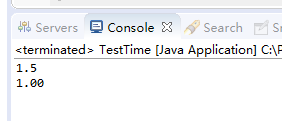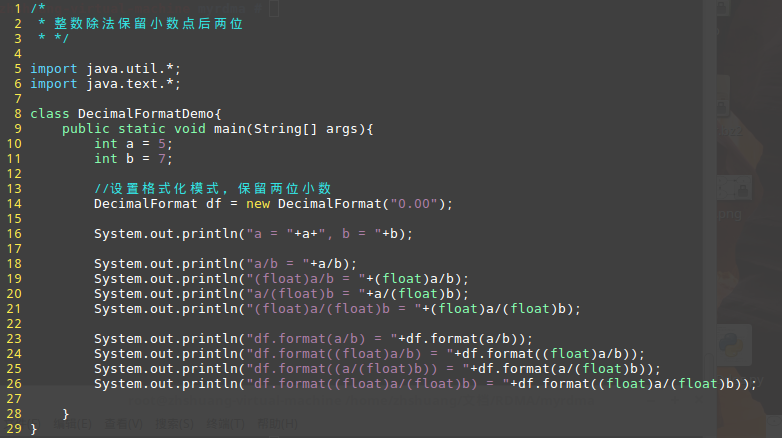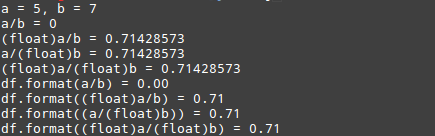• 1.四舍五入保留小数点后四位String.format("%.4f", bmi)2.直接截取小数点后两位，不用进行四舍五入。totalPayment = (int)(totalPayment * 100) / 100.0;
1.四舍五入保留小数点后四位String.format("%.4f", bmi)2.直接截取小数点后两位，不用进行四舍五入。totalPayment = (int)(totalPayment * 100) / 100.0;
展开全文• ##格式时，如果小数点后小于两位，则会原样输出，大于两位时则保留两位小数；使用#.00时，不管小数点后几位，都会保留两位小数。 DecimalFormat df = new DecimalFormat("#.##"); System.out.println(df...
public static void main(String[] args) {		//使用#.##格式时，如果小数点后小于两位，则会原样输出，大于两位时则保留两位小数；使用#.00时，不管小数点后几位，都会保留两位小数。		DecimalFormat df = new DecimalFormat("#.##");		System.out.println(df.format(1.5));		System.out.println(new DecimalFormat("#.00").format(1));	}展开全文• 可以使用DecimalFormat实现保留小数点后两位。 构造函数： DecimalFormat ( String  pattern)  使用给定的模式和默认语言环境的符号创建一个 DecimalFormat。 DecimalFormat ( String  pattern,...
DecimalFormat
DecimalFormat 是 NumberFormat 的一个具体子类，用于格式化十进制数字。该类设计有各种功能，使其能够解析和格式化任意语言环境中的数，包括对西方语言、阿拉伯语和印度语数字的支持。它还支持不同类型的数，包括整数 (123)、定点数 (123.4)、科学记数法表示的数 (1.23E4)、百分数 (12%) 和金额 (\$123)。所有这些内容都可以本地化。
可以使用DecimalFormat实现保留小数点后两位。
构造函数：
DecimalFormat(String pattern)
使用给定的模式和默认语言环境的符号创建一个 DecimalFormat。
DecimalFormat(String pattern,DecimalFormatSymbols symbols)

使用给定的模式和符号创建一个 DecimalFormat。
格式化方法：
StringBufferformat(double number,StringBuffer result,

FieldPosition fieldPosition)
格式化一个 double 值，以生成一个字符串。
StringBufferformat(long number,StringBuffer result,

FieldPosition fieldPosition)
格式化一个 long 值，以生成一个字符串。
StringBufferformat(Object number,StringBuffer toAppendTo,

FieldPosition pos)
格式化一个数，并将所得文本追加到给定的字符串缓冲区。
代码：运行结果：补充：
对于Integer的用法，之前的博客总结过，今天总结一下Integer类的方法valueOf()。

public static Integer valueOf(int i)
返回一个表示指定的 int 值的 Integer 实例。如果不需要新的 Integer 实例，则通常应优先使用该方法，而不是构造方法

Integer(int)，因为该方法有可能通过缓存经常请求的值而显著提高空间和时间性能。

意思是说，如果使用该方法创建对象的话，在内存中如果存在该整数值，那么就不需要重新开辟空间了，类似于将Integer m = n;n在byte的范围内(-128~127)。所以，有如下结果：
Integer n1 = new Integer(1);
Integer n2 = Integer.valueOf(1);
Integer n3 = 1;
System.out.println(n1==n2);//false
System.out.println(n2==n3);//true
在栈内存中有n1，n2，n3，堆内存中有一个对象实体new Integer(1)，n1存储该对象实体的地址，n2指向内存中的数字“1”，定义n3的时候，因为内存中已经有了数字“1”，因此n3直接指向该数字，因此，n1!=n2，n2==n3。

展开全文格式化
• Math.round这个函数的作用是取最接近的int型， ...如果想要两位小数，那就先将原有的数字扩大一百倍，再取整，然后再缩小一百倍，那么结果就是保留两位小数 具体如下： float m = (float) Math.round(d*100)/
Math.round这个函数的作用是取最接近的int型，
如 float d = 123.2323232323;
result = Math.round(d);
那么结果就是 ：123

如果想要两位小数，那就先将原有的数字扩大一百倍，再取整，然后再缩小一百倍，那么结果就是保留了两位小数

具体如下：
float m = (float) Math.round(d*100)/100;
那么m的结果是：123.23


展开全文• 关于保留小数点后几位的问题: ...java保留小数点后两位的问题 在js页面中保留小数点后面两位的方法很简单，就是直接在： 需要转换的小数后面.toFixed(2) 保留几位就给几 但是在控制层保留小数点后面两...
• 保留小数点后两位代码，可以使用java.text包中的DecimalFomart类将指定数字保留两位小数，代码示例如下：java.text.DecimalFormat df =new java.text.DecimalFormat("#.00"); //"#.00" #...
• 在js页面中保留小数点后面两位的方法很简单，就是直接在： 需要转换的小数后面.toFixed(2) 但是在控制层保留小数点后面两位的方法是： new java.text.DecimalFormat("0.00").format(需要转换的小数...
• 1、标准版，保留两位，不会四舍五入 DecimalFormat formater = new DecimalFormat(); formater.setMaximumFractionDigits(2); formater.setGroupingSize(0); formater.setRoundingMode(RoundingMode.FLOOR); ...
• package ... import java.math.BigDecimal; import java.math.RoundingMode; import java.text.DecimalFormat; import java.text.NumberFormat; import java.util.Formatter; /** * @author...
• 一、java.text.DecimalFormat  DecimalFormat df=new DecimalFormat("#.##"); System.out.println(df.format(1.289756)); 二、java.math.BigDecimal BigDecimal bd = new BigDecimal(1.289765); System....小数
• import java.math.BigDecimal; import java.text.DecimalFormat; import java.text.NumberFormat; public class format { double f = 111231.5585; public void m1() { BigDecimal bg = new BigDecimal(f);...
• 方法一：使用DecimalFormat类（最常用） //1.#.00 表示两位小数 #.0000四位小数 以此类推... double d1=123.4567; DecimalFormat df=new DecimalFormat("#.00"); String dd1=df.format(d1); //返回的是一个...
• ## JAVA如何保留小数点后两位

万次阅读 多人点赞 2018-07-21 09:55:42
JAVA如何保留小数点后两位，有其他方法欢迎补充
• 在Android中需要保留小数点后两位，要求精度，查了些资料写成demo 下面是代码：  double d = 3.2865937;  String result = String.format("%.2f", d);  System.out.println(result);    double myNum2 = ...
• 一、Math函数介绍 1）ceil()：将小数部分一律向整数部分进位。Math.ceil(12.2)返回13.0 2）floor()：舍去小数，仅保留整数。Math.floor(12.2)返回12.0 ...二、保留小数点后两位 优先用第二种方式。 1、方式一：四舍
• （1）使用BigDecimal ，保留小数点后两位 public static String format1(double value) { BigDecimal bd = new BigDecimal(value); bd = bd.setScale(2, RoundingMode.HALF_UP); return bd.toString(); } （2）...
• double f = 1314.135645(以保留小数点后两位为例)  方法一：  public void m1( ) {  BigDecimal bg = new BigDecimal(f);  Double f1 = bg.setScale(2,BigDecimal.ROUND_HALF_UP).doubleValue( );
• 一、使用DecimalFormat类 使用DecimalFormat类 （1）、第一种写法 double d = 0.200; DecimalFormat df = new DecimalFormat("0.00"); ...经测试，不管double d的值为多少，最后结果都是正常的两位正则表达式
• JAVA中，有时定义的double数据，在经过运算之后会产生很多小数位，有时只希望保留特定的小数位数，两位三位等等，这里介绍两种方法去实现。。。。
• public class NumberUtils { private NumberUtils() { } public static String doubleToString(Double value) { BigDecimal b = BigDecimal.valueOf(value).setScale(2, BigDecimal.ROUND_HALF_UP);...
• JAVA后端保留小数点后两位的方法1.(double) (Math.round(sd3*10000)/10000.0);这样为保持4位(double) (Math.round(sd3*100)/100.0);这样为保持2位.2.另一种办法import java.text.DecimalFormat;DecimalFormat df2 = ...
• JAVA保留小数点后两位方法： 1.String.format System.out.println(String.format("%.2f", mRepayment)); 2.import java.text.DecimalFormat;  DecimalFormat df = new DecimalFormat("#.00"); df.format(a);......

# java保留小数点后两位java 订阅